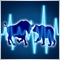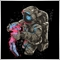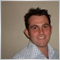EA to trade only on certain time periods - page 241

polymath:

Thanks a lot onewithzachy, Luenbo!  Your suggestions worked perfectly.

I've actually solved this one while waiting for somebody to reply.  I got this idea from the book "Expert Advisor Programming" by Andrew Young:

Just thought of sharing my code in case somebody might stumble across the same task.

Thanks again!

I just have one question to ask. Where should i put this code in order it might work ?10

polymath:

Thanks a lot onewithzachy, Luenbo!  Your suggestions worked perfectly.

I've actually solved this one while waiting for somebody to reply.  I got this idea from the book "Expert Advisor Programming" by Andrew Young:

Just thought of sharing my code in case somebody might stumble across the same task.

Thanks again!

Thank You very much for saving my time! i optimized your code into one function for easier use:

bool checktime(string starttime,string endtime) {
string dt    = TimeToString(TimeCurrent());
string DTstr = TimeToString(TimeCurrent(),TIME_DATE);
string start = DTstr + " " + starttime;
string end   = DTstr + " " + endtime;
StringToTime(start);
StringToTime(end);
StringToTime(dt);

if( start<end  ) if( dt>=start && dt<end ) return(true);
if( start>=end ) if( dt>=start || dt<end ) return(true);
return(false);
}

you should add above code in global scope and use it in OnTick in this way:

if( checktime("02:00:00","22:00:00") )
{
//Do Something ...
}249

Thank You very much for saving my time! i optimized your code into one function for easier use:

you should add above code in global scope and use it in OnTick in this way:

Thank you so much for this, I appreciate the updated function!294

polymath:

Hi onewithzachy,

Thanks for the advise! I've also stumbled across this article:

MQL5 Wizard - Trade Signals Based on Crossover of Two EMA with intraday time filter

but I find it very difficult to understand since all the links to  CSignalITF    and CSignal2EMA_ITF  lead to a 404 error page.

I've resorted to digging up the source codes in the include folder instead and I'm trying to  dissect the classes line by line.

Thanks again!

I had this same problem a few days ago and some one helped me so I will help you.

First of all you need a simple bool funtion

if((Hour() >= 7 && Hour() <= 9) || (Hour() >= 13 && Hour() <= 15))
{
return true;
}
return false;
}

you can see here that I am allowing trades between the hours of 7am and 9am then 1pm and 3 pm.

I then need to call the function just before entering the trade and declare it to be true for the trade to work.

Hope that helpsModerator
18437

you can see here that I am allowing trades between the hours of 7am and 9am then 1pm and 3 pm

I then need to call the function just before entering the trade and declare it to be true for the trade to work.

Hope that helps

if((Hour() >= 7 && Hour() <= 9) || (Hour() >= 13 && Hour() <= 15))
{
return true;
}
return false;
}

You are not.

You are allowing trades between 7AM and 9:59:59 , 1PM and 3:59:5919843

tuxTrader: Thank You very much for saving my time! i optimized your code into one function for easier use:
bool checktime(string starttime,string endtime) {
string dt    = TimeToString(TimeCurrent());
string DTstr = TimeToString(TimeCurrent(),TIME_DATE);
string start = DTstr + " " + starttime;
string end   = DTstr + " " + endtime;
StringToTime(start);
StringToTime(end);
StringToTime(dt);

if( start<end  ) if( dt>=start && dt<end ) return(true);
if( start>=end ) if( dt>=start || dt<end ) return(true);
return(false);
}

This can be optimized:

Not compiled, not tested

bool checktime(string starttime, string endtime) { return checktime(TimeToString(starttime), TimeToString(endtime)); }
bool checktime(datetime start, datetime end) {
datetime dt    = TimeCurrent();
return start<end ? (dt>=start && dt<end) : (dt>=start || dt<end);
}

Not compiled, not tested.